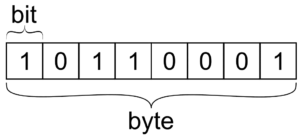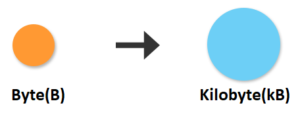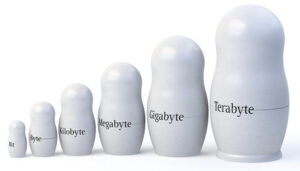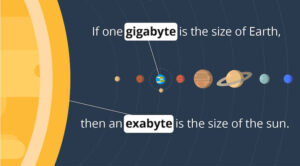# What You Need to Know About Bits, Bytes, and Kilobytes [Dev Concepts #31]

In this article of the series Dev Concepts, we take a look at Digital Units of Measure such as Bits, Bytes, and Kilobytes.For this article, we will take a look at bits, bytes, kilobytes and other digital units in computing. Decimal units such as kilobyte (KB), megabyte (MB), and gigabyte (GB) are commonly used to express the size of data. We will briefly go through them, starting from the smallest unitbit.

As we have said, bits are the smallest unit of data used in computing. A bit is a single unit of data, which takes only two possible values: either zero (0) or one (1).It can store anything, which has two separate states:

• Logical values (true or false). An example of this will be – “is the registration open now or it’s closed“.
• Algebraic signs (plus or minus) – positive or negative number.
• Activation states (on or off) – Similar to the first example – “the lights are switched on” or “the lights are switched off“.

In computer memory, bits don’t stay alone. They are organized in sequences of 8 bits, called bytes. These are the machine “words“. Some devices use 8-bit words, others use 16-bit words, while others use 32-bit words, but usually, bits in memory are accessed in groups (bytes in most systems). That is the reason why the capacity of computer memory is measured in bytes and megabytes (not in bits and megabits).Kilobytes (denoted by KB or KiB) consist of 1024 bytes. In some contexts, 1 kilobyte can mean 1000 bytes (not 1024), which comes from the widely accepted prefix “kilo“, which means 1000. For example, the hard-drive manufacturers use 1000-based kilobytes to measure the hard drive capacity, so have in mind that hard drives are smaller than their label in the shop says.

Megabytes (denoted as MB) consist of 1024 kilobytes, which calculates to 1 048 576 bytes. For example, a photo taken with your smartphone camera is several megabytes of compressed data, holding the image pixels.

Gigabytes (denoted as GB) consist of 1024 megabytes. One gigabyte holds 1 073 741 824 bytes. For example, a 1-hour long video compressed in Full HD quality takes a few gigabytes of storage.

In the same way, the next unit is a terabyte. Terabytes (denoted as TB) consist of 1024 gigabytes. One terabyte holds nearly 1.1 trillion bytes. One terabyte hard drive typically stores a few hundred Full HD movies.The next unit is a terabyte. Terabytes (denoted as TB) consist of 1024 gigabytes. One terabyte holds nearly 1.1 trillion bytes. One terabyte hard drive typically stores a few hundred Full HD movies.Petabytes (denoted as PB) consist of 1024 terabytes. One petabyte holds nearly 1.13 quadrillions of bytes. Modern data centers provide and manage storage with a capacity of multiple petabytes, sometimes multiple exabytes. The next units after petabyte are exabyte, zettabyte, and yottabyte.

In computing, a unit of information is the capacity of some standard data storage system used to measure the capacities of other systems. The most commonly used units of data storage capacity are the bit, the capacity of a system, and the byte, which is equivalent to 8 bits.

## Lesson Topics

In this tutorial we cover the following topics:
• Bits

• Bit, Byte, KB, MB, GB, TB, and PB